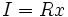# Principal ideal

This article defines a property of an ideal in a commutative unital ring |View other properties of ideals in commutative unital rings
This property of ideals in commutative unital rings depends only on the ideal, viewed abstractly as a module over the commutative unital ring. The corresponding module property that the ideal must satisfy is: cyclic module
View other such properties

## Definition

### Symbol-free definition

An ideal in a commutative unital ring is termed a principal ideal if it is the ideal generated by a single element of the ring.

### Definition with symbols

An ideal$I$ in a ring$R$ is termed a principal ideal if there exists an$x$ in$R$ such that$I = Rx$.

## Metaproperties

### Trimness

This property of ideals in commutative unital rings is trim: it is satisfied by the zero ideal and by the whole ring, as an ideal of itself

The whole ring, as well as the zero ideal, are principal ideals.

### Intersection-closedness

This property of ideals in commutative unital rings is not closed under taking arbitrary intersections; in other words, an arbitrary intersection of ideals with this property need not have this property

An intersection of principal ideals need not be a principal ideal. However, for a unique factorization domain, it is true that an arbitrary intersection of principal ideals is principal. Thus, given any ideal, there exists a smallest principal ideal containing it.

For a gcd domain, it is true that given any finitely generated ideal, there exists a smallest principal ideal containing it.

The property of being a principal ideal is not closed under taking finite, or arbitrary sums, of ideals. A finite sum of principal ideals is a finitely generated ideal, and every finitely generated ideal is principal iff the ring is a Bezout ring. Any ideal can be expressed as an arbitrary sum of principal ideals, and so an arbitrary sum of principal ideals is a principal ideal iff the ring is a principal ideal ring.